学习率调整
周期性学习率调整
为了使得梯度下降方法能够逃离局部最小值或鞍点，一种经验性的方式是在训练过程中周期性地增大学习率。虽然增加学习率可能短期内有损网络的收敛稳定性，但从长期来看有助于找到更好的局部最优解。一般而言，当一个模型收敛一个平坦（Flat）的局部最小值时，其鲁棒性会更好，即微小的参数变动不会剧烈影响模型能力；而当模型收敛到一个尖锐（Sharp）的局部最小值时，其鲁棒性也会比较差。具备良好泛化能力的模型通常应该是鲁棒的，因此理想的局部最小值应该是平坦的。周期性学习率调整可以使得梯度下降方法在优化过程中跳出尖锐的局部极小值，虽然会短期内会损害优化过程，但最终会收敛到更加理想的局部极小值。下面介绍两种常用的周期性调整学习的方法。
循环学习率
循环学习率 一种简单的方法是使用循环学习率（Cyclic Learning Rate）[Accurate, large mini-batch sgd: Training imagenet in 1 hour，Goyal. et al., 2017]，即在让学习率在一个区间内周期性地增大和缩小。通常可以使用线性缩放来调整学习率，称为三角循环学习率（Triangular Cyclic Learning Rate）。假设每个循环周期的长度相等都为2∆T，其中前∆T 步为学习率线性增大阶段，后∆T 步为学习率线性缩小阶段。在第t次迭代时，其所在的循环周期数m为其中⌊·⌋表示“向下取整”函数。第t次迭代的学习率为其中αmax,αmin分别为第m个周期中学习率的上界和下界，可以随着m的增大而逐渐降低；b ∈ [0,1]的计算为带热重启的随机梯度下降
带热重启的随机梯度下降（Stochastic Gradient Descent with Warm Restarts，SGDR）[Loshchilov and Hutter, 2017a] 是用热重启方式来替代学习率衰减的方法。学习率每间隔一定周期后重新初始化为某个预先设定值，然后逐渐衰减。每次重启后模型参数不是从头开始优化，而是从重启前的参数基础上继续优化。 假设在梯度下降过程中重启M次，第m次重启在上次重启开始第Tm个回合后进行，Tm称为重启周期。在第m次重启之前，采用余弦衰减来降低学习率。第t次迭代的学习率为其中α max ,αmin 分别为第m个周期中学习率的上界和下界，可以随着m的增大而逐渐降低；Tcur 为从上次重启之后的回合（Epoch）数。Tcur 可以取小数，比如0.1,0.2等，这样可以在一个回合内部进行学习率衰减。重启周期Tm 可以随着重启次数逐渐增加，比如Tm = Tm−1× κ，其中κ ≥ 1为放大因子。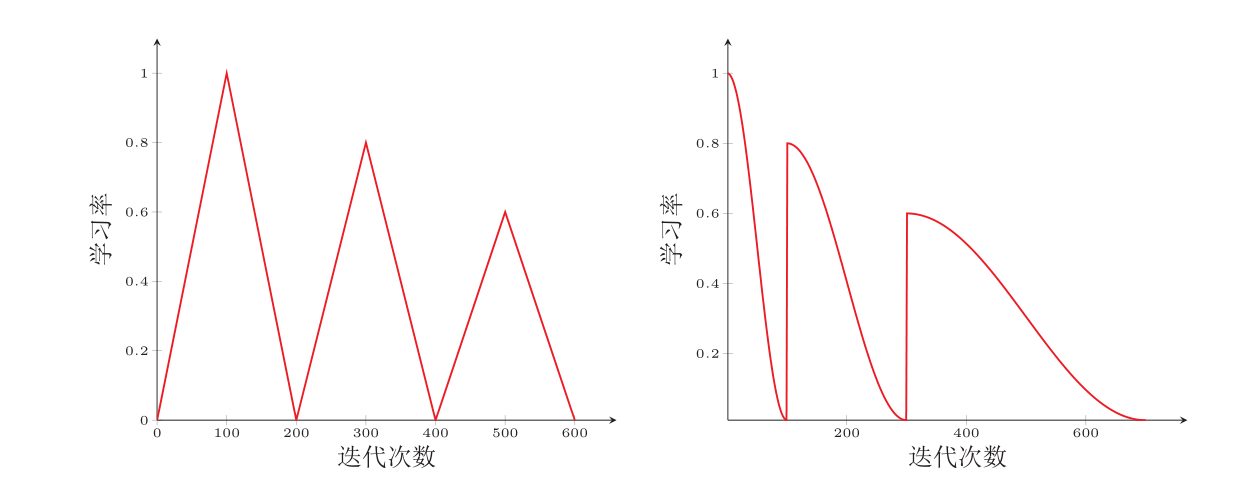(1) 三角循环学习率 (2) 带热重启的余弦衰减 上图为两种周期性学习率调整的示例（假设初始学习率为1），每个周期中学习率的上界也逐步衰减。
RMSprop算法
分类
更新方向优化
除了调整学习率之外，还可以通过使用最近一段时间内的平均梯度来代替当前时刻的梯度来作为参数更新的方向。在小批量梯度下降中，如果每次选取样本数量比较小，损失会呈现震荡的方式下降。有效地缓解梯度下降中的震荡的方式是通过用梯度的移动平均来代替每次的实际梯度，并提高优化速度，这就是动量法。
动量法
动量是模拟物理中的概念。一般而言，一个物体的动量指的是这个物体在它运动方向上保持运动的趋势，是物体的质量和速度的乘积。动量法（MomentumMethod）[Rumelhart et al., 1988]是用之前积累动量来替代真正的梯度。每次迭代的梯度可以看作是加速度。 在第t次迭代时，计算负梯度的“加权移动平均”作为参数的更新方向，其中ρ为动量因子，通常设为0.9，α为学习率。这样，每个参数的实际更新差值取决于最近一段时间内梯度的加权平均值。当某个参数在最近一段时间内的梯度方向不一致时，其真实的参数更新幅度变小；相反，当在最近一段时间内的梯度方向都一致时，其真实的参数更新幅度变大，起到加速作用。一般而言，在迭代初期，梯度方向都比较一致，动量法会起到加速作用，可以更快地到达最优点。在迭代后期，梯度方向会取决不一致，在收敛值附近震荡，动量法会起到减速作用，增加稳定性。从某种角度来说，当前梯度叠加上部分的上次梯度，一定程度上可以近似看作二阶梯度。
分类


展开全文人工智能
• 在训练神经网络时，使用学习率控制参数的更新速度．学习率较小时，会大大降低参数的更新速度；学习率较大时，会使搜索过程中发生震荡，导致参数在极优值附近徘徊． 为此，在训练过程中引入学习...学习率衰减代码学习。
• ## Tensorflow实现各种学习率衰减

万次阅读 多人点赞 2018-02-18 12:45:35
Tensorflow实现学习率衰减 觉得有用的话,欢迎一起讨论相互学习~Follow Me 参考文献 Deeplearning AI Andrew Ng Tensorflow1.2 API 学习率衰减(learning rate decay) 加快学习算法的一个办法就是随...
Tensorflow实现各种学习率衰减
觉得有用的话,欢迎一起讨论相互学习~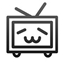参考文献Deeplearning AI Andrew NgTensorflow1.2 API

学习率衰减(learning rate decay)
加快学习算法的一个办法就是随时间慢慢减少学习率,我们将之称为学习率衰减(learning rate decay)
概括
假设你要使用mini-batch梯度下降法,mini-batch数量不大,大概64或者128个样本,但是在迭代过程中会有噪音,下降朝向这里的最小值,但是不会精确的收敛,所以你的算法最后在附近摆动.,并不会真正的收敛.因为你使用的是固定的 α\alpha,在不同的mini-batch中有杂音,致使其不能精确的收敛.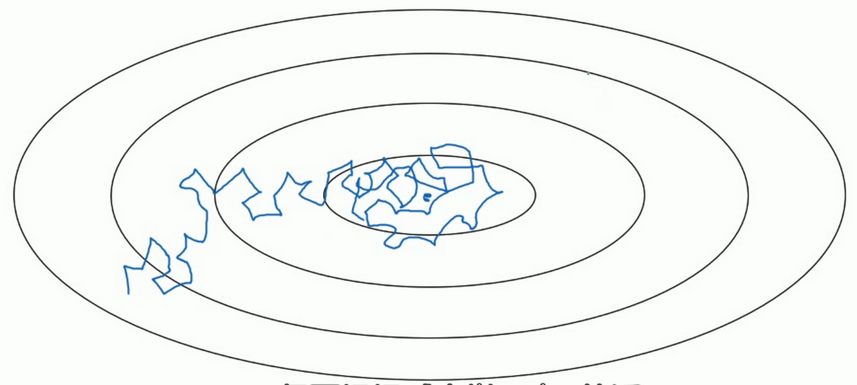但如果能慢慢减少学习率 α\alpha 的话,在初期的时候,你的学习率还比较大,能够学习的很快,但是随着 α\alpha 变小,你的步伐也会变慢变小.所以最后的曲线在最小值附近的一小块区域里摆动.所以慢慢减少 α\alpha 的本质在于在学习初期,你能承受较大的步伐, 但当开始收敛的时候,小一些的学习率能让你的步伐小一些.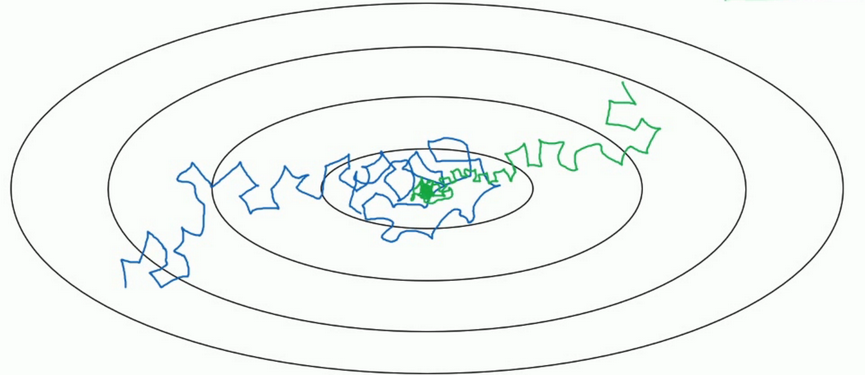细节
一个epoch表示要遍历一次数据,即就算有多个mini-batch,但是一定要遍历所有数据一次,才叫做一个epoch.
学习率 α,其中α0表示初始学习率,decay−rate是一个新引入的超参数\alpha ,其中 \alpha_{0}表示初始学习率, decay-rate是一个新引入的超参数 :α=11+decay−rate∗epoch−num∗α0\alpha = \frac{1}{1+decay-rate*epoch-num}*\alpha_{0}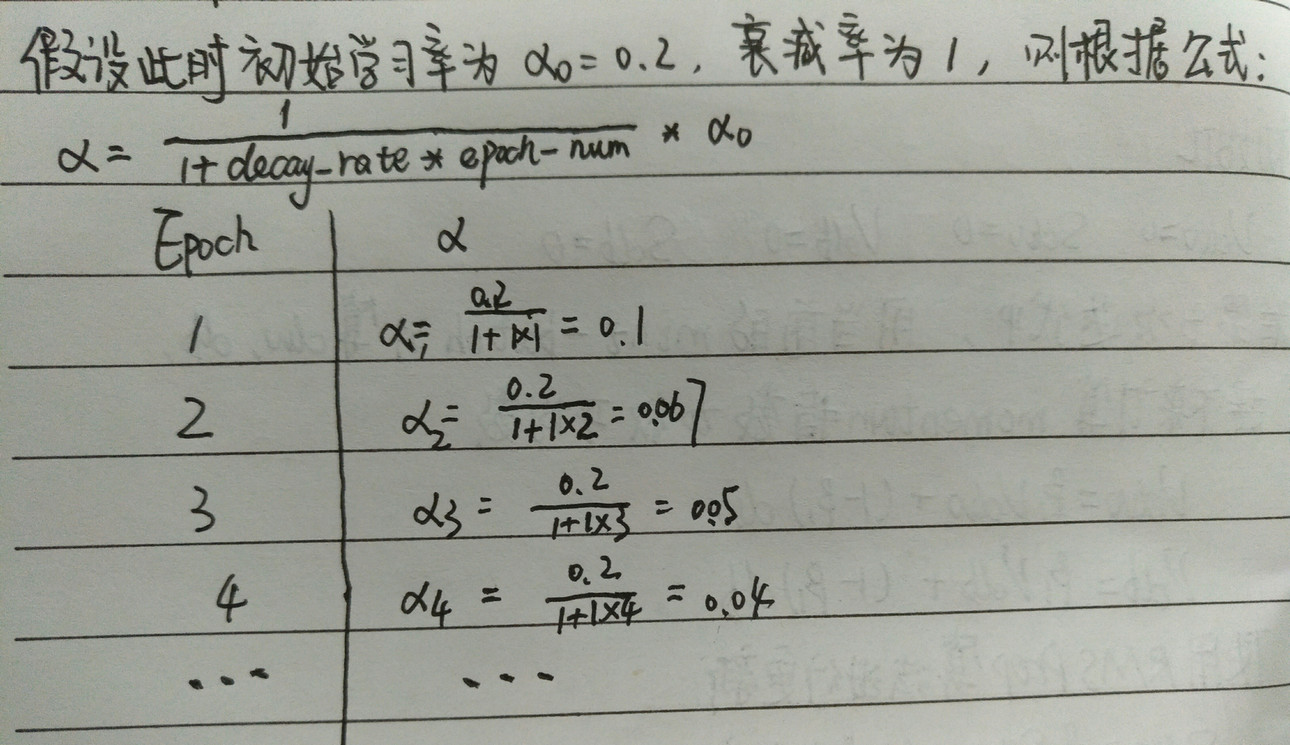其他学习率是衰减公式
指数衰减
α=decay−rateepoch−num∗α0\alpha = decay-rate^{epoch-num}*\alpha_{0}α=kepoch−num∗α0其中k是超参数\alpha = \frac{k}{\sqrt{epoch-num}}*\alpha_{0}其中k是超参数α=kt∗α0其中k是超参数,t表示mini−batch的标记数字\alpha = \frac{k}{\sqrt{t}}*\alpha_{0}其中k是超参数,t表示mini-batch的标记数字
Tensorflow实现学习率衰减
自适应学习率衰减
tf.train.exponential_decay(learning_rate, global_step, decay_steps, decay_rate, staircase=False, name=None)
退化学习率,衰减学习率,将指数衰减应用于学习速率。
计算公式:
decayed_learning_rate = learning_rate * decay_rate ^ (global_step / decay_steps)
# 初始的学习速率是0.1，总的迭代次数是1000次，如果staircase=True，那就表明每decay_steps次计算学习速率变化，更新原始学习速率，
# 如果是False，那就是每一步都更新学习速率。红色表示False，蓝色表示True。
import tensorflow as tf
import numpy as np
import matplotlib.pyplot as plt

learning_rate = 0.1  # 初始学习速率时0.1
decay_rate = 0.96  # 衰减率
global_steps = 1000  # 总的迭代次数
decay_steps = 100  # 衰减次数

global_ = tf.Variable(tf.constant(0))
c = tf.train.exponential_decay(learning_rate, global_, decay_steps, decay_rate, staircase=True)
d = tf.train.exponential_decay(learning_rate, global_, decay_steps, decay_rate, staircase=False)

T_C = []
F_D = []

with tf.Session() as sess:
for i in range(global_steps):
T_c = sess.run(c, feed_dict={global_: i})
T_C.append(T_c)
F_d = sess.run(d, feed_dict={global_: i})
F_D.append(F_d)

plt.figure(1)
plt.plot(range(global_steps), F_D, 'r-')# "-"表示折线图,r表示红色,b表示蓝色
plt.plot(range(global_steps), T_C, 'b-')
# 关于函数的值的计算0.96^(3/1000)=0.998
plt.show()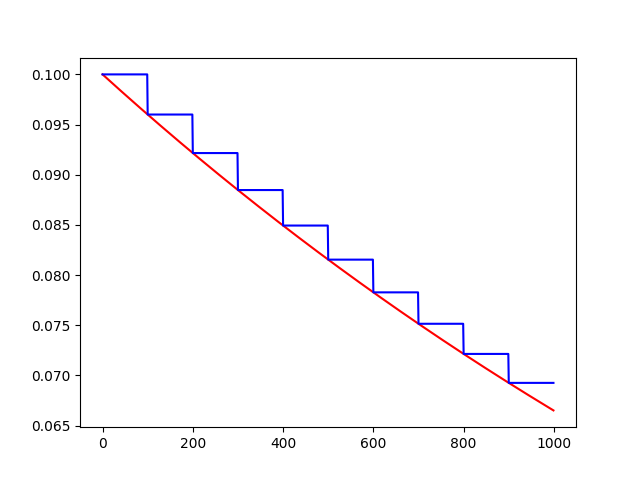反时限学习率衰减
inverse_time_decay(learning_rate, global_step, decay_steps, decay_rate,staircase=False,name=None)
将反时限衰减应用到初始学习率。
计算公式：
decayed_learning_rate = learning_rate / (1 + decay_rate * t)
import tensorflow as tf
import matplotlib.pyplot as plt

global_ = tf.Variable(tf.constant(0), trainable=False)
globalstep = 10000  # 全局下降步数
learning_rate = 0.1  # 初始学习率
decaystep = 1000  # 实现衰减的频率
decay_rate = 0.5  # 衰减率

t = tf.train.inverse_time_decay(learning_rate, global_, decaystep, decay_rate, staircase=True)
f = tf.train.inverse_time_decay(learning_rate, global_, decaystep, decay_rate, staircase=False)

T = []
F = []

with tf.Session() as sess:
for i in range(globalstep):
t_ = sess.run(t, feed_dict={global_: i})
T.append(t_)
f_ = sess.run(f, feed_dict={global_: i})
F.append(f_)

plt.figure(1)
plt.plot(range(globalstep), T, 'r-')
plt.plot(range(globalstep), F, 'b-')
plt.show()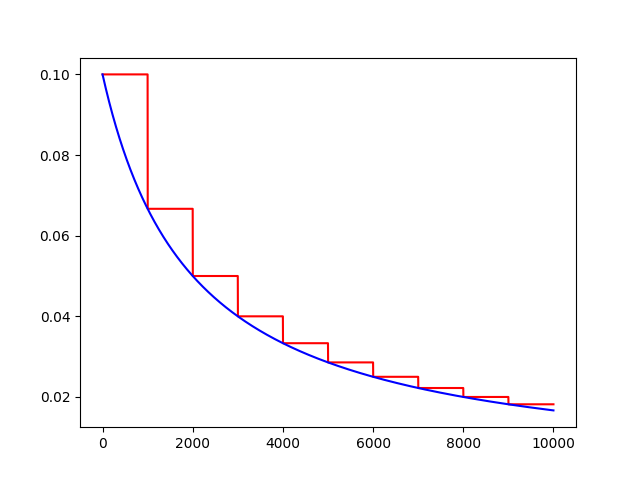学习率自然指数衰减
def natural_exp_decay(learning_rate, global_step, decay_steps, decay_rate, staircase=False, name=None)
将自然指数衰减应用于初始学习速率。
计算公式:
decayed_learning_rate = learning_rate * exp(-decay_rate * global_step)
import tensorflow as tf
import matplotlib.pyplot as plt

global_ = tf.Variable(tf.constant(0), trainable=False)
globalstep = 10000  # 全局下降步数
learning_rate = 0.1  # 初始学习率
decaystep = 1000  # 实现衰减的频率
decay_rate = 0.5  # 衰减率

t = tf.train.natural_exp_decay(learning_rate, global_, decaystep, decay_rate, staircase=True)
f = tf.train.natural_exp_decay(learning_rate, global_, decaystep, decay_rate, staircase=False)

T = []
F = []

with tf.Session() as sess:
for i in range(globalstep):
t_ = sess.run(t, feed_dict={global_: i})
T.append(t_)
f_ = sess.run(f, feed_dict={global_: i})
F.append(f_)

plt.figure(1)
plt.plot(range(globalstep), T, 'r-')
plt.plot(range(globalstep), F, 'b-')
plt.show()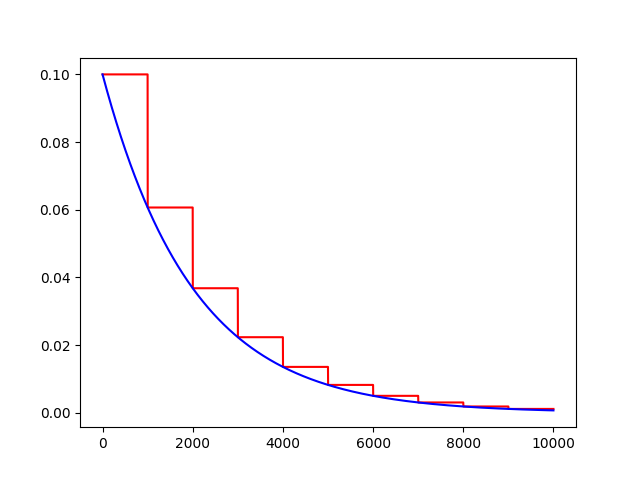常数分片学习率衰减
piecewise_constant(x, boundaries, values, name=None)
例如前1W轮迭代使用1.0作为学习率，1W轮到1.1W轮使用0.5作为学习率，以后使用0.1作为学习率。
import tensorflow as tf
import numpy as np
import matplotlib.pyplot as plt

# 当global_取不同的值时learning_rate的变化，所以我们把global_
global_ = tf.Variable(tf.constant(0), trainable=False)
boundaries = [10000, 12000]
values = [1.0, 0.5, 0.1]
learning_rate = tf.train.piecewise_constant(global_, boundaries, values)
global_steps = 20000

T_L = []
with tf.Session() as sess:
for i in range(global_steps):
T_l = sess.run(learning_rate, feed_dict={global_: i})
T_L.append(T_l)

plt.figure(1)
plt.plot(range(global_steps), T_L, 'r-')
plt.show()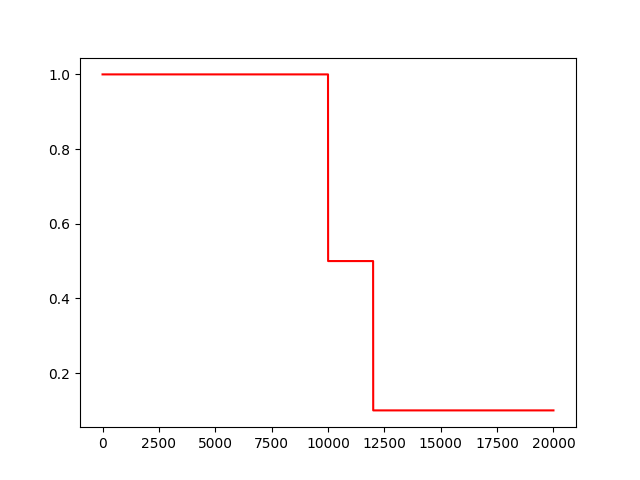多项式学习率衰减
特点是确定结束的学习率。
polynomial_decay(learning_rate, global_step, decay_steps,end_learning_rate=0.0001, power=1.0,cycle=False, name=None):
通常观察到，通过仔细选择的变化程度的单调递减的学习率会产生更好的表现模型。此函数将多项式衰减应用于学习率的初始值。
使学习率learning_rate在给定的decay_steps中达到end_learning_rate。它需要一个global_step值来计算衰减的学习速率。你可以传递一个TensorFlow变量，在每个训练步骤中增加global_step = min(global_step, decay_steps)
计算公式:
decayed_learning_rate = (learning_rate - end_learning_rate) *(1 - global_step / decay_steps) ^ (power) + end_learning_rate
如果cycle为True，则使用decay_steps的倍数，第一个大于’global_steps.ceil表示向上取整.
decay_steps = decay_steps * ceil(global_step / decay_steps)
decayed_learning_rate = (learning_rate - end_learning_rate) *(1 - global_step / decay_steps) ^ (power) + end_learning_rate
Example: decay from 0.1 to 0.01 in 10000 steps using sqrt (i.e. power=0.5):'''
import tensorflow as tf
import matplotlib.pyplot as plt

global_ = tf.Variable(tf.constant(0), trainable=False)
starter_learning_rate = 0.1  # 初始学习率
end_learning_rate = 0.01  # 结束学习率
decay_steps = 1000
globalstep = 10000
f = tf.train.polynomial_decay(starter_learning_rate, global_, decay_steps, end_learning_rate, power=0.5, cycle=False)
t = tf.train.polynomial_decay(starter_learning_rate, global_, decay_steps, end_learning_rate, power=0.5, cycle=True)
F = []
T = []
with tf.Session() as sess:
for i in range(globalstep):
f_ = sess.run(f, feed_dict={global_: i})
F.append(f_)
t_ = sess.run(t, feed_dict={global_: i})
T.append(t_)

plt.figure(1)
plt.plot(range(globalstep), F, 'r-')
plt.plot(range(globalstep), T, 'b-')
plt.show()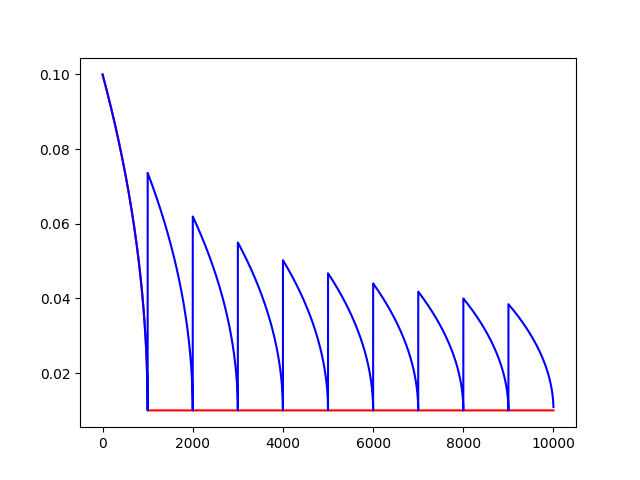展开全文Tensorflow
• ## 学习率衰减方法

千次阅读 2019-06-24 00:29:37
学习率衰减，是每次迭代衰减的方法，在前向运算中。 学习率设定思路是前期一个较大的学习率来确保加速收敛，后期用较小的学习率保证稳定。一般都是0.01,0.005,0.0001等等之类的，设置初始值。 指数衰减 固定...
目录

指数衰减

固定值衰减

按步长衰减

给定值衰减

优化器优化的是学习率，他发生在反向运算中。学习率衰减，是每次迭代衰减的方法，在前向运算中。

学习率设定思路是前期一个较大的学习率来确保加速收敛，后期用较小的学习率保证稳定。一般都是0.01,0.005,0.0001等等之类的，设置初始值。

指数衰减

固定值衰减

按步长衰减

基础学习率。小于1衰减率。stepsize是个阈值，当前迭代步伐step除以他，向下取整作为的指数。衰减系数和stepsize都是经验值。

给定值衰减

facenet中有个文件 ，设定迭代多少次用某个学习率。

还有按倒数衰减，按多项式衰减，都需要经验系数来确定，如果经验不足，可以设置自适应。

学习率衰减 总结

				版权声明：本文为博主原创文章，未经博主允许不得转载。					https://blog.csdn.net/akadiao/article/details/79560731				</div>
<div id="content_views" class="markdown_views">
<!-- flowchart 箭头图标 勿删 -->
<svg xmlns="http://www.w3.org/2000/svg" style="display: none;"><path stroke-linecap="round" d="M5,0 0,2.5 5,5z" id="raphael-marker-block" style="-webkit-tap-highlight-color: rgba(0, 0, 0, 0);"></path></svg>
<h3 id="学习率衰减"><a name="t0"></a>学习率衰减</h3>


学习率衰减（learning rate decay）
在训练神经网络时，使用学习率控制参数的更新速度．学习率较小时，会大大降低参数的更新速度；学习率较大时，会使搜索过程中发生震荡，导致参数在极优值附近徘徊．
为此，在训练过程中引入学习率衰减，使学习率随着训练的进行逐渐衰减．

TensorFlow中实现的学习率衰减方法：

tf.train.piecewise_constant　分段常数衰减
tf.train.inverse_time_decay　反时限衰减
tf.train.polynomial_decay　多项式衰减
tf.train.exponential_decay　指数衰减
tf.train.natural_exp_decay　自然指数衰减
tf.train.cosine_decay　余弦衰减
tf.train.linear_cosine_decay　线性余弦衰减
tf.train.noisy_linear_cosine_decay　噪声线性余弦衰减
函数返回衰减的学习率．
分段常数衰减

tf.train.piecewise_constant()　指定间隔的分段常数．
参数：

x：0-D标量Tensor．
boundaries：边界，tensor或list.
values：指定定义区间的值．
name：操作的名称，默认为PiecewiseConstant．
分段常数衰减就是在定义好的区间上，分别设置不同的常数值，作为学习率的初始值和后续衰减的取值．

示例：

#!/usr/bin/python
# coding:utf-8

# piecewise_constant 阶梯式下降法
import matplotlib.pyplot as plt
import tensorflow as tf
global_step = tf.Variable(0, name='global_step', trainable=False)
boundaries = [10, 20, 30]
learing_rates = [0.1, 0.07, 0.025, 0.0125]
y = []
N = 40
with tf.Session() as sess:
sess.run(tf.global_variables_initializer())
for global_step in range(N):
learing_rate = tf.train.piecewise_constant(global_step, boundaries=boundaries, values=learing_rates)
lr = sess.run([learing_rate])
y.append(lr)

x = range(N)
plt.plot(x, y, 'r-', linewidth=2)
plt.title('piecewise_constant')
plt.show()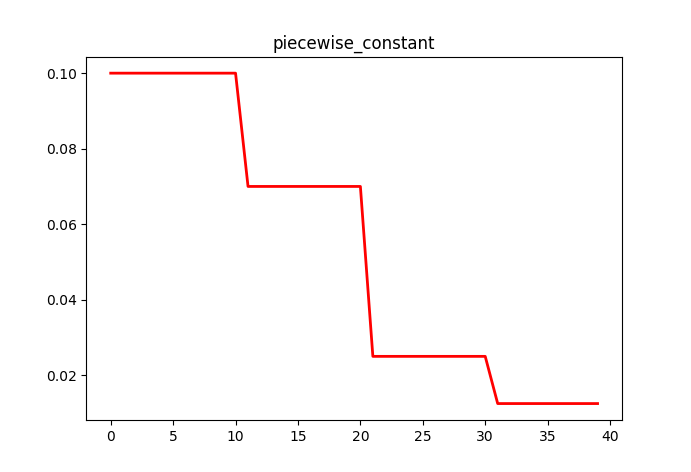指数衰减

指数衰减

tf.train.exponential_decay()　应用指数衰减的学习率．
指数衰减是最常用的衰减方法．
参数：

learning_rate：初始学习率．
global_step：用于衰减计算的全局步数，非负．用于逐步计算衰减指数．
decay_steps：衰减步数，必须是正值．决定衰减周期．
decay_rate：衰减率．
staircase：若为True，则以不连续的间隔衰减学习速率即阶梯型衰减（就是在一段时间内或相同的eproch内保持相同的学习率）；若为False，则是标准指数型衰减．
name：操作的名称，默认为ExponentialDecay．（可选项）
指数衰减的学习速率计算公式为：

decayed_learning_rate = learning_rate * decay_rate ^ (global_step / decay_steps)

优点：简单直接，收敛速度快．

示例，阶梯型衰减与指数型衰减对比：

#!/usr/bin/python
# coding:utf-8
import matplotlib.pyplot as plt
import tensorflow as tf
global_step = tf.Variable(0, name='global_step', trainable=False)

y = []
z = []
N = 200
with tf.Session() as sess:
sess.run(tf.global_variables_initializer())
for global_step in range(N):
# 阶梯型衰减
learing_rate1 = tf.train.exponential_decay(
learning_rate=0.5, global_step=global_step, decay_steps=10, decay_rate=0.9, staircase=True)
# 标准指数型衰减
learing_rate2 = tf.train.exponential_decay(
learning_rate=0.5, global_step=global_step, decay_steps=10, decay_rate=0.9, staircase=False)
lr1 = sess.run([learing_rate1])
lr2 = sess.run([learing_rate2])
y.append(lr1)
z.append(lr2)

x = range(N)
fig = plt.figure()
ax.set_ylim([0, 0.55])
plt.plot(x, y, 'r-', linewidth=2)
plt.plot(x, z, 'g-', linewidth=2)
plt.title('exponential_decay')
ax.set_xlabel('step')
ax.set_ylabel('learing rate')
plt.show()

如图，红色：阶梯型；绿色：指数型：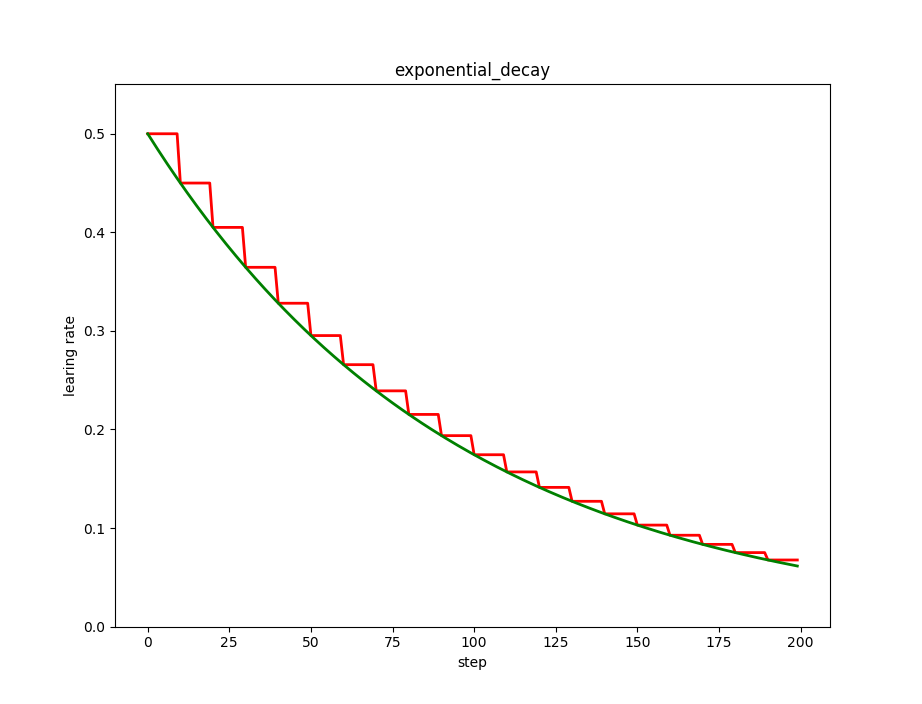自然指数衰减

tf.train.natural_exp_decay() 　应用自然指数衰减的学习率．
参数：

learning_rate：初始学习率．
global_step：用于衰减计算的全局步数，非负.
decay_steps：衰减步数．
decay_rate：衰减率．
staircase：若为True，则是离散的阶梯型衰减（就是在一段时间内或相同的eproch内保持相同的学习率）；若为False，则是标准型衰减．
name: 操作的名称，默认为ExponentialTimeDecay．
natural_exp_decay 和 exponential_decay 形式近似，natural_exp_decay的底数是e．自然指数衰减比指数衰减要快的多，一般用于较快收敛，容易训练的网络．
自然指数衰减的学习率计算公式为：

decayed_learning_rate = learning_rate * exp(-decay_rate * global_step)

示例，指数衰减与自然指数衰减的阶梯型与指数型：

#!/usr/bin/python
# coding:utf-8

import matplotlib.pyplot as plt
import tensorflow as tf
global_step = tf.Variable(0, name='global_step', trainable=False)

y = []
z = []
w = []
N = 200
with tf.Session() as sess:
sess.run(tf.global_variables_initializer())
for global_step in range(N):
# 阶梯型衰减
learing_rate1 = tf.train.natural_exp_decay(
learning_rate=0.5, global_step=global_step, decay_steps=10, decay_rate=0.9, staircase=True)
# 标准指数型衰减
learing_rate2 = tf.train.natural_exp_decay(
learning_rate=0.5, global_step=global_step, decay_steps=10, decay_rate=0.9, staircase=False)
# 指数衰减
learing_rate3 = tf.train.exponential_decay(
learning_rate=0.5, global_step=global_step, decay_steps=10, decay_rate=0.9, staircase=False)
lr1 = sess.run([learing_rate1])
lr2 = sess.run([learing_rate2])
lr3 = sess.run([learing_rate3])
y.append(lr1)
z.append(lr2)
w.append(lr3)

x = range(N)
fig = plt.figure()
ax.set_ylim([0, 0.55])
plt.plot(x, y, 'r-', linewidth=2)
plt.plot(x, z, 'g-', linewidth=2)
plt.plot(x, w, 'b-', linewidth=2)
plt.title('natural_exp_decay')
ax.set_xlabel('step')
ax.set_ylabel('learing rate')
plt.show()

如图，红色：阶梯型；绿色：指数型；蓝色指数型衰减：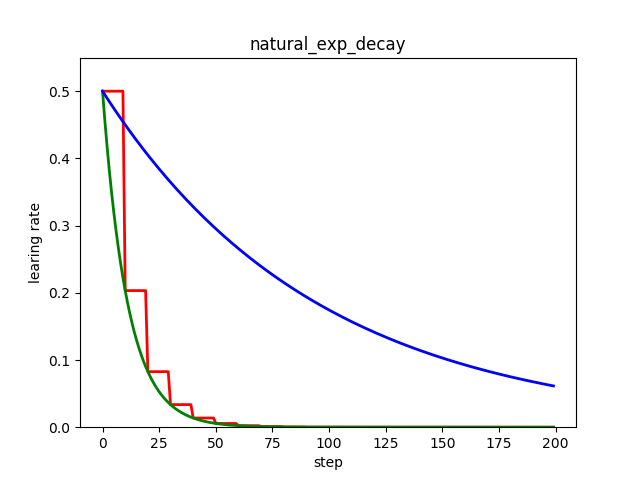多项式衰减

tf.train.polynomial_decay()　应用多项式衰减的学习率．
参数：

learning_rate：初始学习率．
global_step：用于衰减计算的全局步数，非负.
decay_steps：衰减步数，必须是正值．
end_learning_rate：最低的最终学习率．
power：多项式的幂，默认为1.0（线性）．
cycle：学习率下降后是否重新上升．
name：操作的名称，默认为PolynomialDecay。
函数使用多项式衰减，以给定的decay_steps将初始学习率（learning_rate）衰减至指定的学习率（end_learning_rate）．

多项式衰减的学习率计算公式为：

global_step = min(global_step,decay_steps)
decayed_learning_rate = (learning_rate-end_learning_rate)*(1-global_step/decay_steps)^ (power)+end_learning_rate

参数cycle决定学习率是否在下降后重新上升．若cycle为True，则学习率下降后重新上升；使用decay_steps的倍数，取第一个大于global_steps的结果．

decay_steps = decay_steps*ceil(global_step/decay_steps)
decayed_learning_rate = (learning_rate-end_learning_rate)*(1-global_step/decay_steps)^ (power)+end_learning_rate

参数cycle目的：防止神经网络训练后期学习率过小导致网络一直在某个局部最小值中振荡；这样，通过增大学习率可以跳出局部极小值．

示例，学习率下降后是否重新上升对比：

#!/usr/bin/python
# coding:utf-8
# 学习率下降后是否重新上升
import matplotlib.pyplot as plt
import tensorflow as tf
y = []
z = []
N = 200
global_step = tf.Variable(0, name='global_step', trainable=False)

with tf.Session() as sess:
sess.run(tf.global_variables_initializer())
for global_step in range(N):
# cycle=False
learing_rate1 = tf.train.polynomial_decay(
learning_rate=0.1, global_step=global_step, decay_steps=50,
end_learning_rate=0.01, power=0.5, cycle=False)
# cycle=True
learing_rate2 = tf.train.polynomial_decay(
learning_rate=0.1, global_step=global_step, decay_steps=50,
end_learning_rate=0.01, power=0.5, cycle=True)
lr1 = sess.run([learing_rate1])
lr2 = sess.run([learing_rate2])
y.append(lr1)
z.append(lr2)

x = range(N)
fig = plt.figure()
plt.plot(x, z, 'g-', linewidth=2)
plt.plot(x, y, 'r--', linewidth=2)
plt.title('polynomial_decay')
ax.set_xlabel('step')
ax.set_ylabel('learing rate')
plt.show()

如图，红色：下降后不再上升；绿色：下降后重新上升：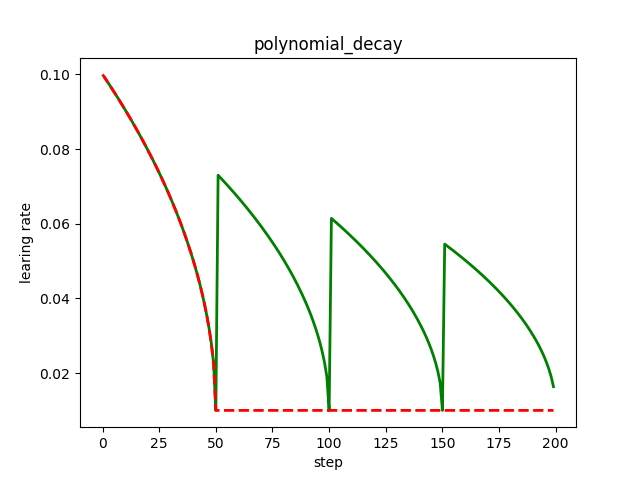余弦衰减

余弦衰减

tf.train.cosine_decay()　将余弦衰减应用于学习率
参数：

learning_rate：标初始学习率．
global_step：用于衰减计算的全局步数.
decay_steps：衰减步数．
alpha：最小学习率（learning_rate的部分）。
name：操作的名称，默认为CosineDecay．
根据论文SGDR: Stochastic Gradient Descent with Warm Restarts提出．

余弦衰减的学习率计算公式为：

global_step = min(global_step, decay_steps)
cosine_decay = 0.5 * (1 + cos(pi * global_step / decay_steps))
decayed = (1 - alpha) * cosine_decay + alpha
decayed_learning_rate = learning_rate * decayed

线性余弦衰减

tf.train.linear_cosine_decay()　将线性余弦衰减应用于学习率．
参数：

learning_rate：标初始学习率．
global_step：用于衰减计算的全局步数.
decay_steps：衰减步数。
num_periods：衰减余弦部分的周期数．
alpha：见计算．
beta：见计算．
name：操作的名称，默认为LinearCosineDecay。
根据论文Neural Optimizer Search with Reinforcement Learning提出．

线性余弦衰减的学习率计算公式为：

global_step=min(global_step,decay_steps)
linear_decay=(decay_steps-global_step)/decay_steps)
cosine_decay = 0.5*(1+cos(pi*2*num_periods*global_step/decay_steps))
decayed=(alpha+linear_decay)*cosine_decay+beta
decayed_learning_rate=learning_rate*decayed

噪声线性余弦衰减

tf.train.noisy_linear_cosine_decay()　将噪声线性余弦衰减应用于学习率．
参数：

learning_rate：标初始学习率．
global_step：用于衰减计算的全局步数.
decay_steps：衰减步数．
initial_variance：噪声的初始方差．
variance_decay：衰减噪声的方差．
num_periods：衰减余弦部分的周期数．
alpha：见计算．
beta：见计算．
name：操作的名称，默认为NoisyLinearCosineDecay．
根据论文Neural Optimizer Search with Reinforcement Learning提出．在衰减过程中加入了噪声，一定程度上增加了线性余弦衰减的随机性和可能性．

噪声线性余弦衰减的学习率计算公式为：

global_step=min(global_step,decay_steps)
linear_decay=(decay_steps-global_step)/decay_steps)
cosine_decay=0.5*(1+cos(pi*2*num_periods*global_step/decay_steps))
decayed=(alpha+linear_decay+eps_t)*cosine_decay+beta
decayed_learning_rate =learning_rate*decayed

示例，线性余弦衰减与噪声线性余弦衰减：

#!/usr/bin/python
# coding:utf-8
import matplotlib.pyplot as plt
import tensorflow as tf
y = []
z = []
w = []
N = 200
global_step = tf.Variable(0, name='global_step', trainable=False)

with tf.Session() as sess:
sess.run(tf.global_variables_initializer())
for global_step in range(N):
# 余弦衰减
learing_rate1 = tf.train.cosine_decay(
learning_rate=0.1, global_step=global_step, decay_steps=50,
alpha=0.5)
# 线性余弦衰减
learing_rate2 = tf.train.linear_cosine_decay(
learning_rate=0.1, global_step=global_step, decay_steps=50,
num_periods=0.2, alpha=0.5, beta=0.2)
# 噪声线性余弦衰减
learing_rate3 = tf.train.noisy_linear_cosine_decay(
learning_rate=0.1, global_step=global_step, decay_steps=50,
initial_variance=0.01, variance_decay=0.1, num_periods=0.2, alpha=0.5, beta=0.2)
lr1 = sess.run([learing_rate1])
lr2 = sess.run([learing_rate2])
lr3 = sess.run([learing_rate3])
y.append(lr1)
z.append(lr2)
w.append(lr3)

x = range(N)
fig = plt.figure()
plt.plot(x, z, 'b-', linewidth=2)
plt.plot(x, y, 'r-', linewidth=2)
plt.plot(x, w, 'g-', linewidth=2)
plt.title('cosine_decay')
ax.set_xlabel('step')
ax.set_ylabel('learing rate')
plt.show()

如图，红色：余弦衰减；蓝色：线性余弦衰减；绿色：噪声线性余弦衰减；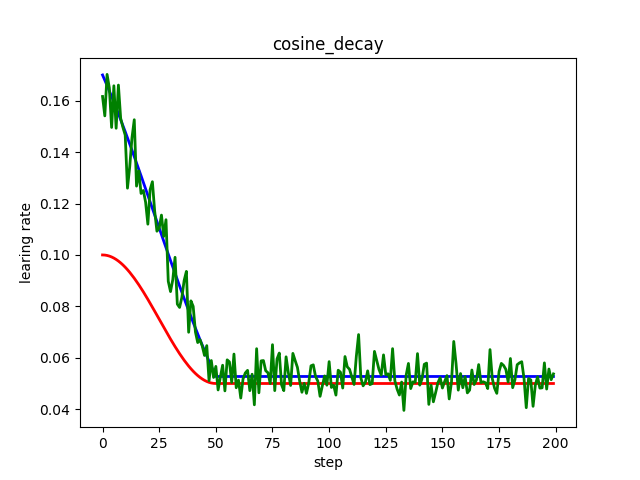反时限衰减

tf.train.inverse_time_decay()　将反时限衰减应用到初始学习率．
参数：

learning_rate：初始学习率．
global_step：用于衰减计算的全局步数．
decay_steps：衰减步数．
decay_rate：衰减率．
staircase：是否应用离散阶梯型衰减．（否则为连续型）
name：操作的名称，默认为InverseTimeDecay．
该函数应用反向衰减函数提供初始学习速率．利用global_step来计算衰减的学习速率．计算公式为：

decayed_learning_rate =learning_rate/(1+decay_rate* global_step/decay_step)

若staircase为True时：

decayed_learning_rate =learning_rate/(1+decay_rate*floor(global_step/decay_step))

示例，反时限衰减的阶梯型衰减与连续型对比：

#!/usr/bin/python
# coding:utf-8

import matplotlib.pyplot as plt
import tensorflow as tf
y = []
z = []
N = 200
global_step = tf.Variable(0, name='global_step', trainable=False)

with tf.Session() as sess:
sess.run(tf.global_variables_initializer())
for global_step in range(N):
# 阶梯型衰减
learing_rate1 = tf.train.inverse_time_decay(
learning_rate=0.1, global_step=global_step, decay_steps=20,
decay_rate=0.2, staircase=True)
# 连续型衰减
learing_rate2 = tf.train.inverse_time_decay(
learning_rate=0.1, global_step=global_step, decay_steps=20,
decay_rate=0.2, staircase=False)
lr1 = sess.run([learing_rate1])
lr2 = sess.run([learing_rate2])

y.append(lr1)
z.append(lr2)

x = range(N)
fig = plt.figure()
plt.plot(x, z, 'r-', linewidth=2)
plt.plot(x, y, 'g-', linewidth=2)
plt.title('inverse_time_decay')
ax.set_xlabel('step')
ax.set_ylabel('learing rate')
plt.show()

如图，蓝色：阶梯型；红色：连续型：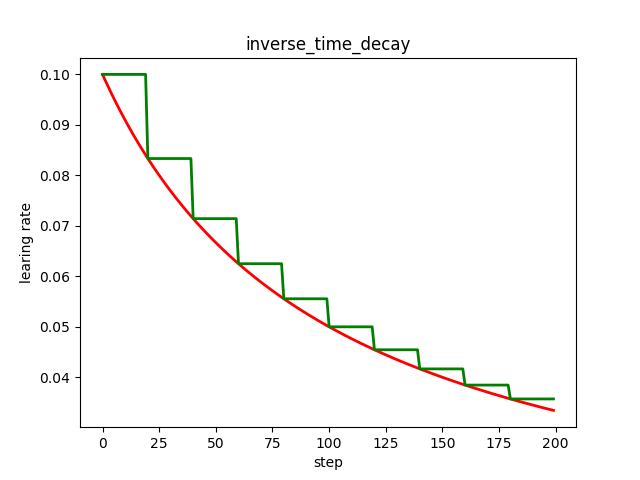展开全文• 01—学习率衰减首先我们以一个例子，来说明一下我们为什么需要学习率α衰减(learning rate decay)。如果学习率不衰减的话，如下图蓝线所示，由于噪音影响，代价函数更新路径相对不规则，但总体朝着最低点方向移动，...


之前我们的优化，主要是聚焦于对梯度下降运动方向的调整，而在参数迭代更新的过程中，除了梯度，还有一个重要的参数是学习率α，对于学习率的调整也是优化的一个重要方面。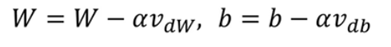01—学习率衰减首先我们以一个例子，来说明一下我们为什么需要学习率α衰减(learning rate decay)。如果学习率不衰减的话，如下图蓝线所示，由于噪音影响，代价函数更新路径相对不规则，但总体朝着最低点方向移动，但是移动到最低点附近时，由于学习率较大，每一次会移动相对较远的距离，容易直接跨过最低点，导致代价函数在更新完毕后距离最低点仍然相对较远；当我们随着迭代的次数逐渐降低学习率，那么便如绿线所示，一开始学习率较大，前进速度较快，到达最低点附近后，学习率降低到更小的值，于是最终更新完成后代价函数离最低点更近，也就是模型更加优化，预测值与实际值差距更小。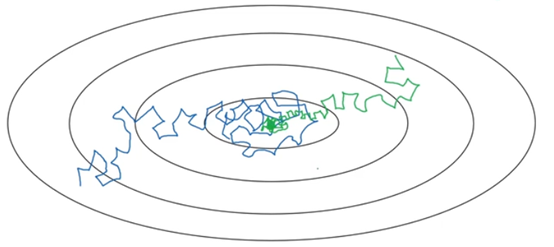进行学习率衰减的一种方式如下所示，需要设置如下学习率更新规则：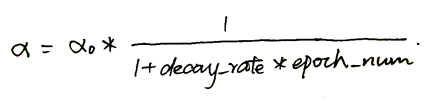1个epoch是指将所有mini-batch全部迭代一遍，即遍历一遍。假设α0=0.2，decay_rate=1，那么随着epoch增加，学习率α会如下图变化：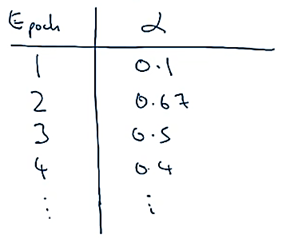在应用这个公式时，我们需要选择合适的超参数α0和decay_rate。除了这种学习率衰减方式，还有一些其他方式来进行学习率衰减：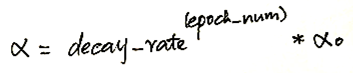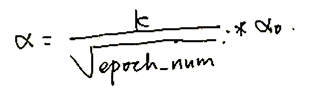此外还有离散衰减，经过一段时间衰减一半：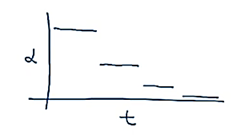02—学习率衰减的pytorch实现指数衰减我们首先需要确定需要针对哪个优化器执行学习率动态调整策略，也就是首先定义一个优化器：optimizer_ExpLR = torch.optim.SGD(net.parameters(), lr=0.1)定义好优化器以后，就可以给这个优化器绑定一个指数衰减学习率控制器：ExpLR = torch.optim.lr_scheduler.ExponentialLR(optimizer_ExpLR, gamma=0.98)参数gamma表示衰减的底数，也就是decay_rate，选择不同的gamma值可以获得幅度不同的衰减曲线。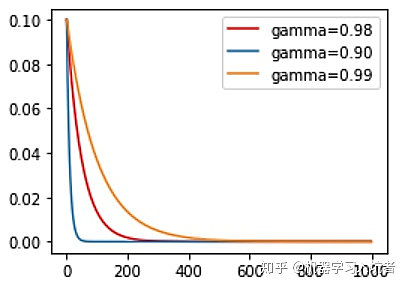固定步长衰减即离散型衰减，学习率每隔一定步数(或者epoch)就减少为原来的gamma分之一，使用固定步长衰减依旧先定义优化器，再给优化器绑定StepLR对象：optimizer_StepLR = torch.optim.SGD(net.parameters(), lr=0.1)StepLR = torch.optim.lr_scheduler.StepLR(optimizer_StepLR, step_size=step_size, gamma=0.65)其中gamma参数表示衰减的程度，step_size参数表示每隔多少个step进行一次学习率调整，下面对比了不同gamma值下的学习率变化情况：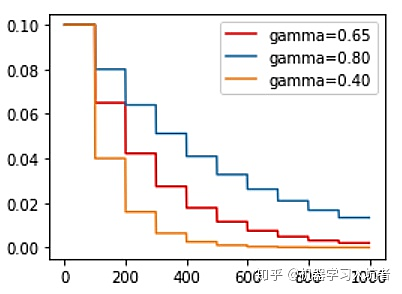多步长衰减有时我们希望不同的区间采用不同的更新频率，或者是有的区间更新学习率，有的区间不更新学习率，这就需要使用MultiStepLR来实现动态区间长度控制：optimizer_MultiStepLR = torch.optim.SGD(net.parameters(), lr=0.1)torch.optim.lr_scheduler.MultiStepLR(optimizer_MultiStepLR,\                milestones=[200, 300, 320, 340, 200], gamma=0.8)其中milestones参数为表示学习率更新的起止区间，在区间[0. 200]内学习率不更新，而在[200, 300]、[300, 320].....[340, 400]的右侧值都进行一次更新；gamma参数表示学习率衰减为上次的gamma分之一。其图示如下：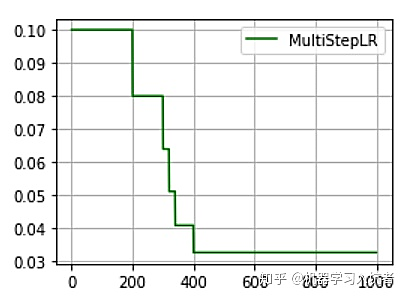从图中可以看出，学习率在区间[200， 400]内快速的下降，这就是milestones参数所控制的，在milestones以外的区间学习率始终保持不变。余弦退火衰减严格的说，余弦退火策略不应该算是学习率衰减策略，因为它使得学习率按照周期变化，其定义方式如下：optimizer_CosineLR = torch.optim.SGD(net.parameters(), lr=0.1)CosineLR = torch.optim.lr_scheduler.CosineAnnealingLR(optimizer_CosineLR, T_max=150, eta_min=0)参数T_max表示余弦函数周期；eta_min表示学习率的最小值，默认它是0表示学习率至少为正值。确定一个余弦函数需要知道最值和周期，其中周期就是T_max，最值是初试学习率。下图展示了不同周期下的余弦学习率更新曲线：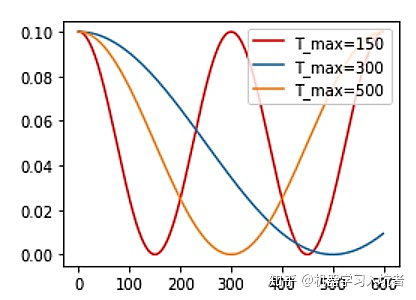为网络的不同层设置不同的学习率定义一个简单的网络结构：class net(nn.Module):    def __init__(self):        super(net, self).__init__()        self.conv1 = nn.Conv2d(3, 64, 1)        self.conv2 = nn.Conv2d(64, 64, 1)        self.conv3 = nn.Conv2d(64, 64, 1)        self.conv4 = nn.Conv2d(64, 64, 1)        self.conv5 = nn.Conv2d(64, 64, 1)    def forward(self, x):        out = conv5(conv4(conv3(conv2(conv1(x)))))        return out我们希望conv5学习率是其他层的100倍，我们可以：net = net()lr = 0.001conv5_params = list(map(id, net.conv5.parameters())) # 1 base_params = filter(lambda p: id(p) not in conv5_params,                     net.parameters()) # 2,3optimizer = torch.optim.SGD([            {'params': base_params},            {'params': net.conv5.parameters(), 'lr': lr * 100}], lr=lr, momentum=0.9)1. conv5_params = list(map(id,net.conv5.parameters()))中id()函数用于获取网络参数的内存地址，map()函数用于将id()函数作用于net.conv5.parameters()得到的每个参数上。2.lambda p: id(p)中lamda表达式是python中用于定义匿名函数的方式，其后面定义的是一个函数操作，冒号前的符号是函数的形式参数，用于接收参数，符合的个数表示需要接收的参数个数，冒号右边是具体的函数操作。3.filter()函数的作用是过滤掉不符合条件(False)的元素，返回一个迭代器对象。该函数接收两个参数，第一个为函数，第二个为序列，序列的每个元素作为参数传递给函数进行判断，然后返回True或False，最后返回值为True的元素Reference深度学习课程 --吴恩达https://zhuanlan.zhihu.com/p/93624972
`
展开全文• 学习率衰减 加快神经网络的另一个办法就是随着时间推移慢慢减少学习率。我们将这个称之为学习率衰减。 如图中蓝色线所示，当mini-batch不大的时候，噪声会比较明显。最后不会精确地收敛，而是在最小值附近不断地...
• 1.学习率衰减的函数 2.导入数据 3.定义模型 4.定义loss和acc 5.定义优化器 6.优化模型 7.结论 【学习率衰减，是因为学习率太大，开始震荡了，因此降低学习率，可以加速收敛】 1.学习率衰减的函数 2.导入...
• 训练前期学习率大，可以加快收敛速度，训练后期仍然使用较大的学习...本文介绍一些 tensorflow 常用的学习率衰减方法。 1、指数衰减 def exponential_decay( learning_rate, # 初始学习率 global_step, # 当前...tensorflow
• 本文转载关于pytorch优化器学习率和学习率衰减的文章： https://blog.csdn.net/ys1305/article/details/94332643 pytorch优化器API和学习率以及学习衰减率API。 ... ...
• pytorch实现学习率衰减 文章目录pytorch实现学习率衰减手动修改optimizer中的lr使用lr_schedulerLambdaLR——lambda函数衰减StepLR——阶梯式衰减MultiStepLR——多阶梯式衰减ExponentialLR——指数连续衰减...
• Tensorflow实现学习率衰减 觉得有用的话,欢迎一起讨论相互学习~Follow Me 参考文献 Deeplearning AI Andrew Ng Tensorflow1.2 API 学习率衰减(learning rate decay) 加快学习算法的一个办法就是随时间慢慢减少学习...
• 1、权重衰减和学习率衰减的理解  2、学习率衰减的不同形式
• 学习率衰减(learning rate decay) 为了防止学习率过大，在收敛到全局最优点的时候会来回摆荡，所以要让学习率随着训练轮数不断按指数级下降，收敛梯度下降的学习步长。 学习率衰减可以用以下代码实现 decayed_...人工智能 TensorFlow
• 深度学习中的固定学习率衰减策略总结深层神经网络的参数学习主要是通过梯度下降方法来寻找一组可以最小化结构风险的参数。 在梯度下降中学习率的取值非常关键，如果过大可能不会收敛，过小则收敛速度太慢。通常的...
• 1 学习率衰减 深度学习模型训练过程中，经过一定的epoch之后，模型的性能趋于饱和，此时降低学习率，在小范围内进一步调整模型的参数，可以进一步提升模型的性能。 经过多年的发展，也出现了多种学习率衰减算法，...
• 关于余弦退火学习率衰减策略的详细介绍可以参考文章：学习率衰减之余弦退火(CosineAnnealing）。 代码实现 在 Tensorflow2.0 中自定义学习率衰减策略其实很简单，可以参考文章：Tensorflow2.0学习率衰减详细汇总。 ...tensorflow python
• 2.学习率衰减常用参数有哪些 3.常见衰减函数 3.1分段常数衰减 3.2指数衰减 3.3自然指数衰减 3.4多项式衰减 3.5余弦衰减 1.学习率的作用 ​ 梯度下降法是一个广泛被用来最小化模型误差的参数优化算法。梯度...
• Pytorch的学习率衰减及其用法1. 为什么需要用到学习率衰减？2. Pytorch中学习率衰减的方法3. 使用库函数进行调整3.1 基本说明3.2 等间隔调整学习率 StepLR3.3 多间隔调整学习率 MultiStepLR3.4 指数衰减调整学习率 ...pytorch
• Tensorflow实现学习率衰减 觉得有用的话,欢迎一起讨论相互学习~Follow Me 参考文献 Deeplearning AI Andrew Ng Tensorflow1.2 API 学习率衰减(learning rate decay) 加快学习算法的一个办法就是随时间慢慢...
• 学习率衰减 学习率衰减（learning rate decay） 在训练神经网络时，使用学习率控制参数的更新速度．学习率较小时，会大大降低参数的更新速度；学习率较大时，会使搜索过程中发生震荡，导致参数在极优值附近徘徊． ...tensorflow
• 文章目录深度学习学习率衰减一、学习率衰减意义二、keras实现学习率衰减1. 阶层性下降参考资料： 深度学习学习率衰减 一、学习率衰减意义 模型训练，关于超参数学习率的设置，一般初始设置为较大的值0.1,然后在学习...神经网络...